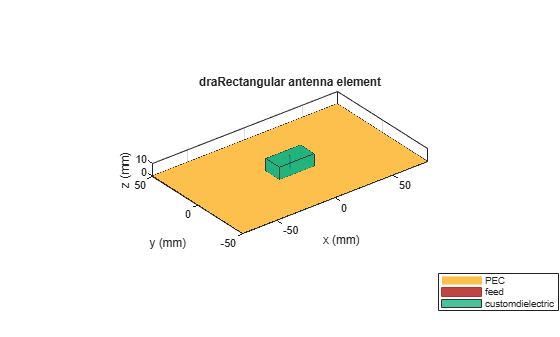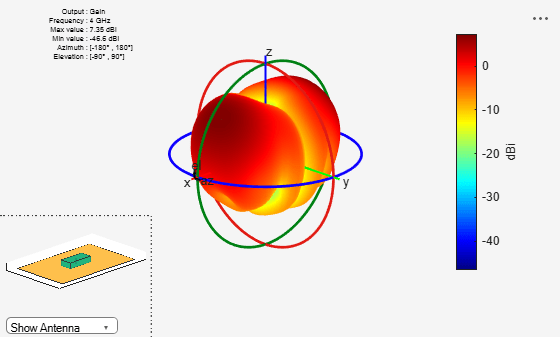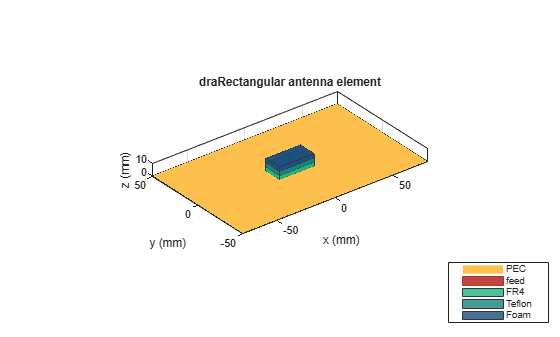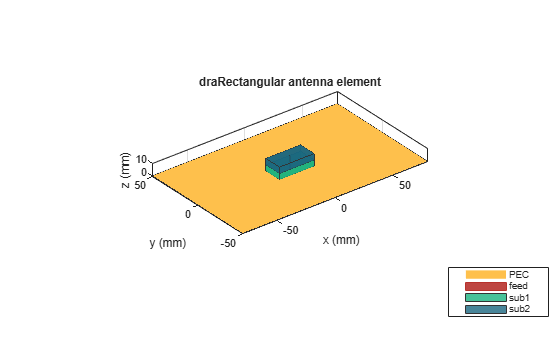# draRectangular

Create rectangular dielectric resonator antenna

Since R2021a

## Description

The `draRectangular` object creates a rectangular dielectric resonator antenna. The rectangular dielectric resonator antenna consists of a rectangular-shaped dielectric placed on a ground plane. It has high power-handling capacity and can provide high gain and bandwidth. The rectangular dielectric resonator antenna has the advantage of two aspect ratio that aids in the generation of various modes. These antennas are more suitable for use at microwave frequencies. Rectangular dielectric resonator antennas are widely used in satellite and radar systems.## Creation

### Syntax

``ant = draRectangular``
``ant = draRectangular(Name=Value)``

### Description

example

````ant = draRectangular` creates a rectangular dielectric resonator antenna with dimensions for a resonant frequency of 3.3 GHz. The default antenna is probe fed with the feed point at the origin.```

example

````ant = draRectangular(Name=Value)` creates a rectangular dielectric resonator antenna with additional Properties specified using one or more name-value arguments. `Name` is the property name and `Value` is the corresponding value. You can specify several name-value pair arguments in any order as `Name1`=`Value1`, `...`, `NameN`=`ValueN`. Properties you do not specify retains their default values.For example, `draRectangular(ResonatorLength=0.04)` creates a rectangular dielectric resonator antenna with the length of the dielectric resonator set to 40 mm.```

## Properties

expand all

Length of the dielectric resonator, specified as a positive scalar in meters.

Example: `0.35`

Data Types: `double`

Width of the dielectric resonator, specified as a positive scalar in meters.

Example: `0.30`

Data Types: `double`

Type of dielectric material used as a substrate, specified as a dielectric object. You can choose any material from the `DielectricCatalog` or specify a dielectric of your choice. For more information, see `dielectric`. For more information on dielectric substrate meshing, see Meshing.

Note

The substrate dimensions must be less than the ground plane dimensions.

Example: `dielectric("FR4")`

Example: ```dielectric(Name="sub1", EpsilonR=2.3, LossTangent=0.002, Thickness=0.01)```

Ground plane length, specified as a positive scalar in meters. By default, ground plane length is measured along the x-axis. Set `GroundPlaneLength` to `Inf` to use the infinite ground plane technique for antenna analysis.

Example: `120e-3`

Data Types: `double`

Ground plane width, specified as a positive scalar in meters. By default, ground plane width is measured along y-axis. Setting `GroundPlaneWidth` to `Inf` to use the infinite ground plane technique for antenna analysis.

Example: `118e-3`

Data Types: `double`

Width of the feed, specified as a positive scalar in meters.

Example: `5e-05`

Data Types: `double`

Height of the feed, specified as a positive scalar in meters.

Example: `0.0050`

Data Types: `double`

Signed distance between feed point and the center of the ground plane, specified as a two-element vector in meters. The two elements represent the distances in x, and y directions from center of the ground plane respectively.

Example: `[–0.0070 0.01]`

Data Types: `double`

Type of the metal used as a conductor, specified as a metal material object. You can choose any metal from the `MetalCatalog` or specify a metal of your choice. For more information, see `metal`. For more information on metal conductor meshing, see Meshing.

Example: `metal("Copper")`

Tilt angle of the antenna in degrees, specified as a scalar or vector. For more information, see Rotate Antennas and Arrays.

Example: `90`

Example: `Tilt=[90 90]`,`TiltAxis=[0 1 0;0 1 1]` tilts the antenna at 90 degrees about the two axes defined by the vectors.

Data Types: `double`

Tilt axis of the antenna, specified as one of these values:

• Three-element vector of Cartesian coordinates in meters. In this case, each coordinate in the vector starts at the origin and lies along the specified points on the x-, y-, and z-axes.

• Two points in space, specified as a 2-by-3 matrix corresponding to two three-element vectors of Cartesian coordinates. In this case, the antenna rotates around the line joining the two points.

• `"x"`, `"y"`, or `"z"` to describe a rotation about the x-, y-, or z-axis, respectively.

Example: `[0 1 0]`

Example: `[0 0 0;0 1 0]`

Example: `"Z"`

Data Types: `double` | `string`

Lumped elements added to the antenna feed, specified as a `lumpedElement` object. You can add a load anywhere on the surface of the antenna. By default, the load is at the feed. For more information, see `lumpedElement`.

Example: `lumpedElement(Impedance=75)`

## Object Functions

 `show` Display antenna, array structures, shapes, or platform `axialRatio` Axial ratio of antenna `beamwidth` Beamwidth of antenna `charge` Charge distribution on antenna or array surface `current` Current distribution on antenna or array surface `design` Design prototype antenna or arrays for resonance around specified frequency or create AI-based antenna from antenna catalog objects `efficiency` Radiation efficiency of antenna `EHfields` Electric and magnetic fields of antennas or embedded electric and magnetic fields of antenna element in arrays `impedance` Input impedance of antenna or scan impedance of array `mesh` Mesh properties of metal, dielectric antenna, or array structure `meshconfig` Change meshing mode of antenna, array, custom antenna, custom array, or custom geometry `optimize` Optimize antenna or array using SADEA optimizer `pattern` Plot radiation pattern and phase of antenna or array or embedded pattern of antenna element in array `patternAzimuth` Azimuth plane radiation pattern of antenna or array `patternElevation` Elevation plane radiation pattern of antenna or array `rcs` Calculate and plot radar cross section (RCS) of platform, antenna, or array `returnLoss` Return loss of antenna or scan return loss of array `sparameters` Calculate S-parameters for antennas and antenna arrays `vswr` Voltage standing wave ratio (VSWR) of antenna or array element

## Examples

collapse all

Create a rectangular dielectric resonator antenna with default properties.

`ant = draRectangular`
```ant = draRectangular with properties: ResonatorLength: 0.0300 ResonatorWidth: 0.0150 Substrate: [1x1 dielectric] GroundPlaneLength: 0.1600 GroundPlaneWidth: 0.1000 FeedWidth: 1.0000e-03 FeedHeight: 0.0200 FeedOffset: [0 0] Conductor: [1x1 metal] Tilt: 0 TiltAxis: [1 0 0] Load: [1x1 lumpedElement] ```

View the antenna using the `show` function.

`show(ant)`Plot the radiation pattern of a rectangular dielectric resonator antenna at a frequency of 4 GHz.

```ant = draRectangular(ResonatorLength=0.045, ResonatorWidth=0.02); pattern(ant,4e9)```Create a rectangular dielectric resonator antenna with FR4, Teflon, and foam as substrates.

``` ant = draRectangular; d = dielectric("FR4","Teflon","Foam"); d.Thickness = [ant.Substrate.Thickness/3 ant.Substrate.Thickness/3 ant.Substrate.Thickness/3]; ant.Substrate = d; ant = draRectangular(Substrate=d); show(ant)```Create a rectangular dielectric resonator antenna with substrates having relative permittivity of 2.3 and 4.5, respectively. The value of loss tangent for both the substrates is 0.002.

```ant = draRectangular; d = dielectric; d.Name = {'sub1','sub2'}; d.EpsilonR = [2.3 4.5]; d.LossTangent = [0.002 0.002]; d.Thickness = [ant.Substrate.Thickness/2 ant.Substrate.Thickness/2]; ant.Substrate = d; show(ant)```